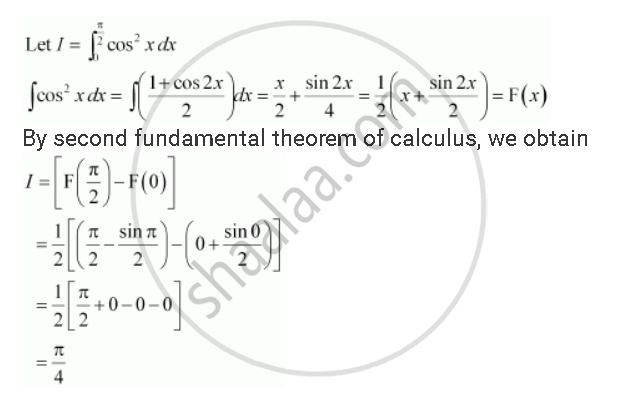Share

# Evaluate the Definite Integrals Int_0^(Pi/2) Cos^2 Xdx - CBSE (Science) Class 12 - Mathematics

#### Question

Evaluate the definite integrals int_0^(pi/2) cos^2 xdx

#### SolutionIs there an error in this question or solution?

#### Video TutorialsVIEW ALL 

Solution Evaluate the Definite Integrals Int_0^(Pi/2) Cos^2 Xdx Concept: Fundamental Theorem of Calculus.
S